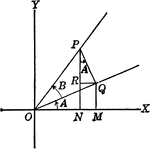### Angles Used to Illustrate Sum and Difference of Two Angles

Angles used to illustrate the sum and difference of two angles and trig identities.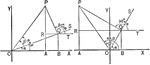### Sum of 2 Acute Angles

Illustration of two triangles, showing the sine of the sum of two acute angles expressed in terms of…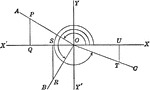### Reference Angles/Triangles Formed by Angles in Quadrants

Lines drawn to horizontal to form triangle ratios (reference triangles, angles).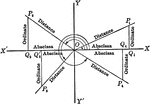### Reference Angles/Triangles Formed by Angles in Quadrants With Labels

Lines drawn to horizontal to form triangle ratios (reference triangles, angles). Axes, quadrants, abscissa,…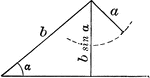### Ambiguous Case

Illustration of one possible outcome (no triangle occurs) when discussing the ambiguous case using the…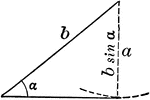### Ambiguous Case

Illustration of one possible outcome (1 triangle occurs) when discussing the ambiguous case using the…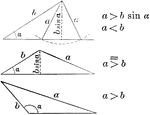### Ambiguous Case

Illustration of one possible outcome (2 triangles occur) when discussing the ambiguous case using the…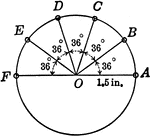### Circle With 36 degree Angles and Radius 1.5 in.

Circle with 36 degree angles marked. This diagram can be used with the following trig problem: Locate…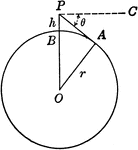### Circle With Center o and Radius r with point P

Circle modeling the earth. O is the center of the earth, r the radius of the earth, and h the height…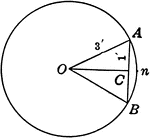### Circle With a Chord of 2 ft. and a Radius of 3 ft.

Circle with chord AB=2 ft. and radius OA = 3 ft.. Triangle AOC is a right triangle. Angle AOC=half angle…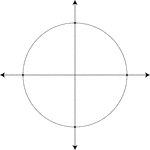### Unit Circle

Illustration of a unit circle (circle with a radius of 1) superimposed on the coordinate plane. The…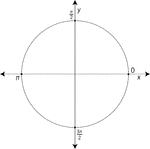### Unit Circle Labeled At Quadrantal Angles

Illustration of a unit circle (circle with a radius of 1) superimposed on the coordinate plane with…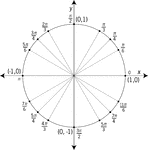### Unit Circle Labeled At Special Angles

Illustration of a unit circle (circle with a radius of 1) superimposed on the coordinate plane with…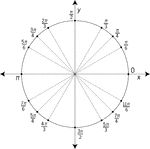### Unit Circle Labeled At Special Angles

Illustration of a unit circle (circle with a radius of 1) superimposed on the coordinate plane with…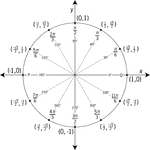### Unit Circle Labeled In 30° Increments With Values

Illustration of a unit circle (circle with a radius of 1) superimposed on the coordinate plane with…### Unit Circle Labeled In 45 ° Increments

Illustration of a unit circle (circle with a radius of 1) superimposed on the coordinate plane with…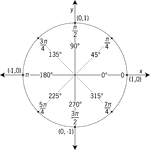### Unit Circle Labeled In 45° Increments With Values

Illustration of a unit circle (circle with a radius of 1) superimposed on the coordinate plane with…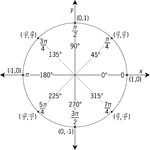### Unit Circle Labeled In 45° Increments With Values

Illustration of a unit circle (circle with a radius of 1) superimposed on the coordinate plane with…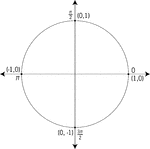### Unit Circle Labeled With Quadrantal Angles And Values

Illustration of a unit circle (circle with a radius of 1) superimposed on the coordinate plane. All…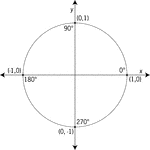### Unit Circle Labeled With Quadrantal Angles And Values

Illustration of a unit circle (circle with a radius of 1) superimposed on the coordinate plane with…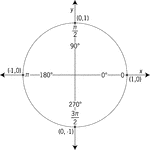### Unit Circle Labeled With Quadrantal Angles And Values

Illustration of a unit circle (circle with a radius of 1) superimposed on the coordinate plane with…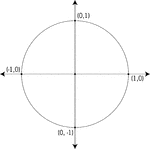### Unit Circle Labeled With Quadrantal Values

Illustration of a unit circle (circle with a radius of 1) superimposed on the coordinate plane. At each…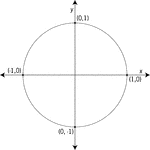### Unit Circle Labeled With Quadrantal Values

Illustration of a unit circle (circle with a radius of 1) superimposed on the coordinate plane with…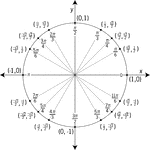### Unit Circle Labeled With Special Angles And Values

Illustration of a unit circle (circle with a radius of 1) superimposed on the coordinate plane with…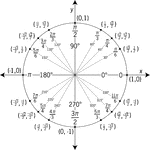### Unit Circle Labeled With Special Angles And Values

Illustration of a unit circle (circle with a radius of 1) superimposed on the coordinate plane with…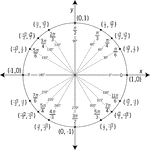### Unit Circle Labeled With Special Angles And Values

Illustration of a unit circle (circle with a radius of 1) superimposed on the coordinate plane with…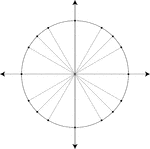### Unit Circle Marked At Special Angles

Illustration of a unit circle (circle with a radius of 1) superimposed on the coordinate plane. All…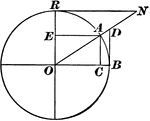### Versedsine and Coversedsine in Circle

The coversedsine is equal to 1 minus the sine. In this figure, AE is parallel to OB; hence, EO = AC…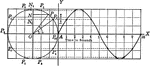### Projection of Points in Circular Motion

Illustration of the projection of point P as it moves around a vertical circle of radius 3 in. in a…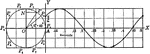### Projection of Points in Circular Motion

Illustration of the projection of point P as it moves around a vertical circle of radius 2 ft. in a…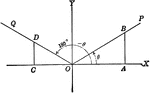### Coordinate Axis With Angles, Lines, and Perpendiculars Drawn

Coordinate axis with angle XOP equal to theta, Θ, and angle XOQ=180 - Θ. From any point in the terminal…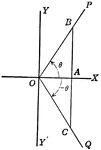### Coordinate Axis With Perpendiculars Drawn To Form Similar Right Triangles From Positive and Negative Theta, Θ

Angle XOP=Θ and angle XOQ=- Θ. From a point in the terminal side of each a perpendicular line is drawn…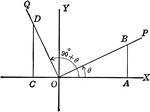### Coordinate Axis With Perpendiculars Drawn To Form Similar Right Triangles

Angle XOP=Θ and angle XOQ=90+Θ. From a point in the terminal side of each a perpendicular line is…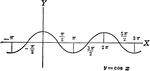### Cosine Curve y=cos x

Cosine curve plotted from negative pi to 2 pi. Graph of y=cos x.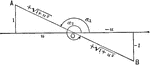### Negative Cotangent Angles

Illustration that can be used to show that if the cotangent of an angle is negative the angle must terminate…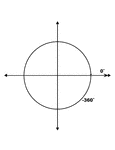### 0° and -360° Coterminal Angles

Illustration showing coterminal angles of 0° and -360°. Coterminal angles are angles drawn in…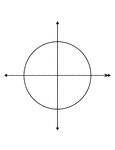### 0° and -360° Coterminal Angles

Illustration showing coterminal angles of 0° and -360°. Coterminal angles are angles drawn in…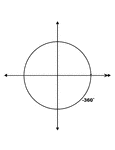### 0° and -360° Coterminal Angles

Illustration showing coterminal angles of 0° and -360°. Coterminal angles are angles drawn in…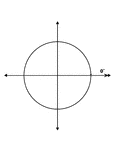### 0° and -360° Coterminal Angles

Illustration showing coterminal angles of 0° and -360°. Coterminal angles are angles drawn in…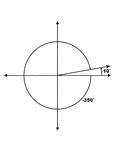### 10° and -350° Coterminal Angles

Illustration showing coterminal angles of 10° and -350°. Coterminal angles are angles drawn…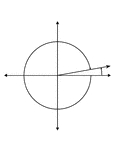### 10° and -350° Coterminal Angles

Illustration showing coterminal angles of 10° and -350°. Coterminal angles are angles drawn…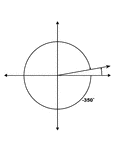### 10° and -350° Coterminal Angles

Illustration showing coterminal angles of 10° and -350°. Coterminal angles are angles drawn…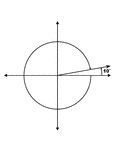### 10° and -350° Coterminal Angles

Illustration showing coterminal angles of 10° and -350°. Coterminal angles are angles drawn…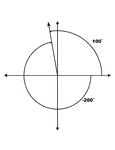### 100° and -260° Coterminal Angles

Illustration showing coterminal angles of 100° and -260°. Coterminal angles are angles drawn…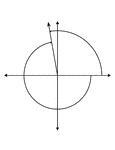### 100° and -260° Coterminal Angles

Illustration showing coterminal angles of 100° and -260°. Coterminal angles are angles drawn…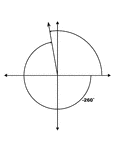### 100° and -260° Coterminal Angles

Illustration showing coterminal angles of 100° and -260°. Coterminal angles are angles drawn…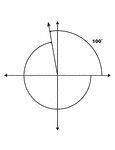### 100° and -260° Coterminal Angles

Illustration showing coterminal angles of 100° and -260°. Coterminal angles are angles drawn…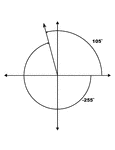### 105° and -255° Coterminal Angles

Illustration showing coterminal angles of 105° and -255°. Coterminal angles are angles drawn…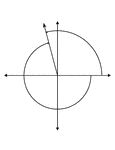### 105° and -255° Coterminal Angles

Illustration showing coterminal angles of 105° and -255°. Coterminal angles are angles drawn…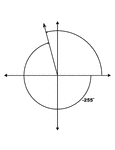### 105° and -255° Coterminal Angles

Illustration showing coterminal angles of 105° and -255°. Coterminal angles are angles drawn…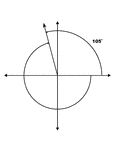### 105° and -255° Coterminal Angles

Illustration showing coterminal angles of 105° and -255°. Coterminal angles are angles drawn…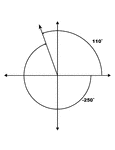### 110° and -250° Coterminal Angles

Illustration showing coterminal angles of 110° and -250°. Coterminal angles are angles drawn…### 110° and -250° Coterminal Angles

Illustration showing coterminal angles of 110° and -250°. Coterminal angles are angles drawn…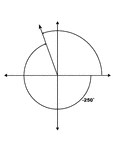### 110° and -250° Coterminal Angles

Illustration showing coterminal angles of 110° and -250°. Coterminal angles are angles drawn…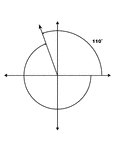### 110° and -250° Coterminal Angles

Illustration showing coterminal angles of 110° and -250°. Coterminal angles are angles drawn…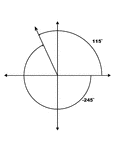### 115° and -245° Coterminal Angles

Illustration showing coterminal angles of 115° and -245°. Coterminal angles are angles drawn…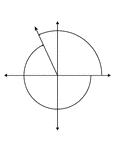### 115° and -245° Coterminal Angles

Illustration showing coterminal angles of 115° and -245°. Coterminal angles are angles drawn…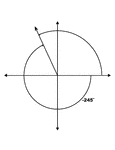### 115° and -245° Coterminal Angles

Illustration showing coterminal angles of 115° and -245°. Coterminal angles are angles drawn…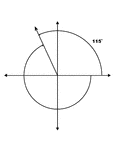### 115° and -245° Coterminal Angles

Illustration showing coterminal angles of 115° and -245°. Coterminal angles are angles drawn…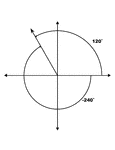### 120° and -240° Coterminal Angles

Illustration showing coterminal angles of 120° and -240°. Coterminal angles are angles drawn…